Updating search results...

# 30 Results

View
Selected filters:
• NC.M1.F-IF.2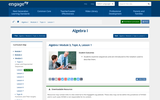Conditional Remix & Share Permitted
CC BY-NC-SA
Rating
0.0 stars
Overview:

Students examine sequences and are introduced to the notation used to describe them.

Subject:
Math 1
Material Type:
Lesson
Author:
EngageNY
02/03/2020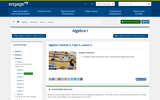Conditional Remix & Share Permitted
CC BY-NC-SA
Rating
0.0 stars
Overview:

Students write sequences with recursive and explicit formulas.

Subject:
Math 1
Material Type:
Lesson
Author:
EngageNY
02/09/2020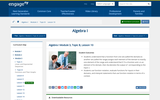Conditional Remix & Share Permitted
CC BY-NC-SA
Rating
0.0 stars
Overview:

Students understand that a function from one set (called the domain) to another set (called the range) assigns each element of the domain to exactly one element of the range and understand that if f is a function and x is an element of its domain, then f(x) denotes the output of corresponding to the input x.
Students use function notation, evaluate functions for inputs in their domains, and interpret statements that use function notation in terms of a context.

Subject:
Math 1
Material Type:
Lesson
Author:
EngageNY
02/09/2020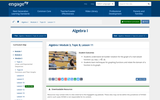Conditional Remix & Share Permitted
CC BY-NC-SA
Rating
0.0 stars
Overview:

Students understand set builder notation for the graph of a real-valued function: {(x, f(x)) | x ∈ D}.
Students learn techniques for graphing functions and relate the domain of a function to its graph.

Subject:
Math 1
Material Type:
Lesson Plan
Author:
EngageNY
02/09/2020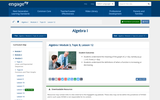Conditional Remix & Share Permitted
CC BY-NC-SA
Rating
0.0 stars
Overview:

Students understand the meaning of the graph of y = f(x), namely {(x,y) | x ∈ D and y = f(x)}.
Students understand the definitions of when a function is increasing or decreasing.

Subject:
Math 1
Material Type:
Lesson
Author:
EngageNY
02/09/2020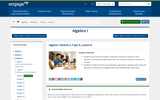Conditional Remix & Share Permitted
CC BY-NC-SA
Rating
0.0 stars
Overview:

Students use function notation, evaluate functions for inputs in their domains, and interpret statements that use function notation in terms of a context.
Students create functions that represent a geometric situation and relate the domain of a function to its graph and to the relationship it describes.

Subject:
Math 1
Material Type:
Lesson
Author:
EngageNY
02/09/2020Conditional Remix & Share Permitted
CC BY-NC-SA
Rating
0.0 stars
Overview:

Students understand that a function from one set (called the domain) to another set (called the range) assigns each element of the domain to exactly one element of the range.
Students use function notation, evaluate functions for inputs in their domains, and interpret statements that use function notation in terms of a context.

Subject:
Math 1
Material Type:
Lesson
Author:
EngageNY
02/09/2020Rating
0.0 stars
Overview:

Worksheet on evaluating functions that have been combined.

Subject:
Mathematics
Material Type:
Activity/Lab
Provider:
Weebly
11/27/2017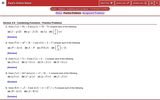Rating
0.0 stars
Overview:

Practice problems combining functions using arithmetic and also composition functions.

Subject:
Mathematics
Material Type:
Activity/Lab
Provider:
Lamar University
11/27/2017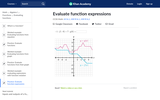Rating
0.0 stars
Overview:

In this Khan Academy activity, students will practice evaluating function expressions given their graph.

Subject:
Mathematics
Material Type:
Activity/Lab
Provider:
08/27/2018Rating
0.0 stars
Overview:

In this Khan Academy activity, students will practice evaluating functions.

Subject:
Mathematics
Material Type:
Activity/Lab
Provider:
08/27/2018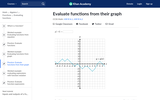Rating
0.0 stars
Overview:

In this Khan Academy activity, students will practice evaluating function expressions given their graph.

Subject:
Mathematics
Material Type:
Activity/Lab
Provider:
08/27/2018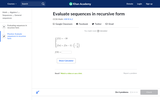Rating
0.0 stars
Overview:

In this Khan Academy activity, students will evaluate sequences in recursive form.

Subject:
Mathematics
Material Type:
Activity/Lab
Provider:
08/27/2018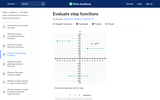Rating
0.0 stars
Overview:

In this Khan Academy activity, students will evaluate step functions.

Subject:
Mathematics
Material Type:
Activity/Lab
Provider:
08/27/2018Rating
0.0 stars
Overview:

This resource provides guided notes about evaluating functions and word problems with practice problems.

Subject:
Mathematics
Material Type:
Activity/Lab
Lesson Plan
Provider:
Lakeview Public Schools
06/25/2018Rating
4.0 stars
Overview:

In this lesson, students will learn to evaluate a function written as an expression by substituting a value into the given expression. Students will also learn that they can substitute another expression into the original expression of the function. They will recognize that g(x) is the output of a function and the expression x is the input. Students will also evaluate functions using tables and graphs.

Subject:
Mathematics
Material Type:
Lesson Plan
Provider:
West Contra Costa Unified
07/02/2018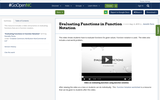Conditional Remix & Share Permitted
CC BY-NC
Rating
0.0 stars
Overview:

This resource includes a video and practice on evaluating functions that are in function notation.

Subject:
Mathematics
Math 1
Material Type:
Formative Assessment
Lecture Notes
Author:
Danielle Flores
11/06/2019
Remix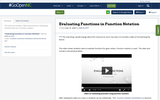Conditional Remix & Share Permitted
CC BY-NC
Rating
0.0 stars
Overview:

This resource includes a video and practice on evaluating functions that are in function notation.

Subject:
Mathematics
Math 1
Material Type:
Formative Assessment
Lecture Notes
Author:
KORI ELLIOTT
07/15/2020Rating
4.0 stars
Overview:

Class activity with scenario where students have to write and evaluate exponential function.

Subject:
Mathematics
Material Type:
Activity/Lab
Provider:
Math from the Hart
07/05/2018Rating
0.0 stars
Overview:

Exponential growth and decay Jeopardy game which encourages students to evaluate exponential problems.

Subject:
Mathematics
Material Type:
Interactive
Provider:
Jeopardy Labs